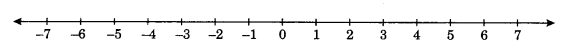On this page, you will find Integers Class 7 Notes Maths Chapter 1 Pdf free download. CBSE NCERT Class 7 Maths Notes Chapter 1 Integers will seemingly help them to revise the important concepts in less time.

## CBSE Class 7 Maths Chapter 1 Notes Integers

### Integers Class 7 Notes Conceptual Facts

1. Number lineFor a positive integer on number line, we move towards right of zero and for a negative integer, we move towards the left of the zero.

2. Integers are closed under addition, subtraction and multiplication.
For example:

• a + b = integer
• a – b = integer
• a x b = integer

3. Addition and multiplication are cumulative for integers.
For example:

• a + b = b + a
• a x b = b x a

4. Addition and multiplication are associative for integers.
For example:

• (a + b) + c = a + (b + c)
• (a x b) x c = a x (b x c)

5. Zero (0) is an additive identity for integers.
For example: a + 0 = 0 + a = a

6. One (1) is a multiplicative identity for integers.
For example: a x 1 = 1 x a = a

7. Algebra of integers,

• a x (-b) = -ab
• (-a) xb = -ab
• (-a) x (-b) = ab
• a xb = ab

8. Product of even number of negative integers is positive whereas the product of odd number of negative integers is negative. For example:

• (-a) x (-b) x (-c) x (-d) (-e) -a x b x c x d x e …. even number times
• (-a) x (-b) x (-c) x (-d) (-e) = -(a x b x c x d x e) odd number times

9. Division on integers:
(i) $$\frac{(-a)}{(b)}=-\left(\frac{a}{b}\right)$$
(ii) $$\frac{(-a)}{(-b)}=\left(\frac{a}{b}\right)$$
(iii) $$\frac{(a)}{(-b)}=-\left(\frac{a}{b}\right)$$
(iv) $$\left(\frac{+a}{+b}\right)=\left(\frac{a}{b}\right)$$